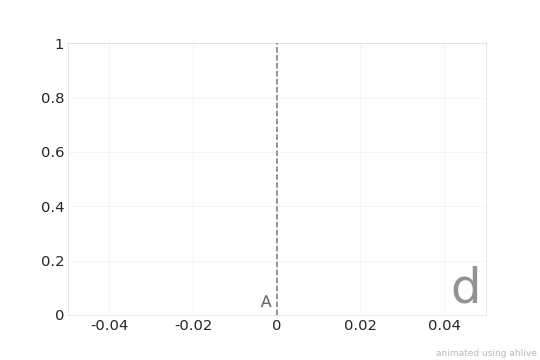# Referencing#

## basic references#

References can be added by passing one or more of x0s, x1s, y0s, y1s.

:

import ahlive as ah
arr = ah.Array([0, 1, 2], [3, 4, 5])
arr = arr.reference(y0s=4)
arr.render()

gifsicle: warning: trivial adaptive palette (only 223 colors in source)

: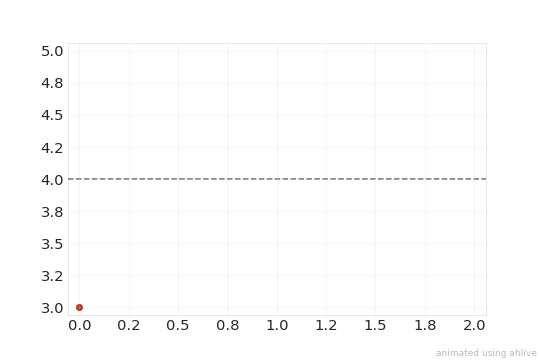Reference values can be animated too if an Iterable is passed.

:

import ahlive as ah
arr = ah.Array([0, 1, 2], [3, 4, 5])
arr = arr.reference(y0s=[3, 3.5, 4.5])
arr.render()

gifsicle: warning: trivial adaptive palette (only 214 colors in source)

: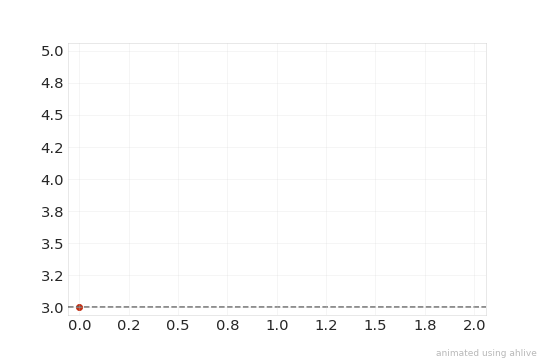## dynamic references#

Like remarks, rather than setting static values for references, passing a label from the dataset, e.g. x, y, c, state_label, and inline_label (without the “s” suffix), can dynamically grab the value for that label. Any labels listed under “Data variables” is valid for use as long as it contains a state dimension.

:

import ahlive as ah
arr = ah.Array([0, 1, 2], [3, 4, 5])
arr = arr.reference(y0s='y')
arr.render()

: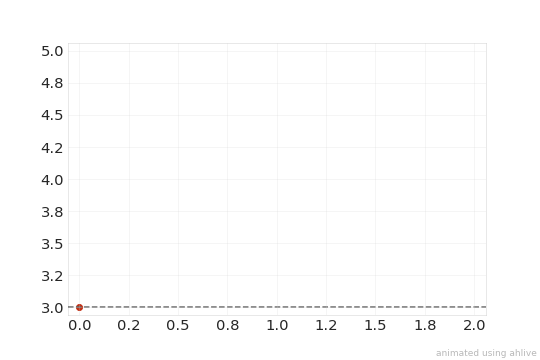For multiple items, last will only plot the last item’s reference.

:

import ahlive as ah
arr = ah.Array([0, 1, 2], [3, 4, 5])
arr = arr - arr
arr = arr.reference(y0s='y', last=True)
arr.render()

: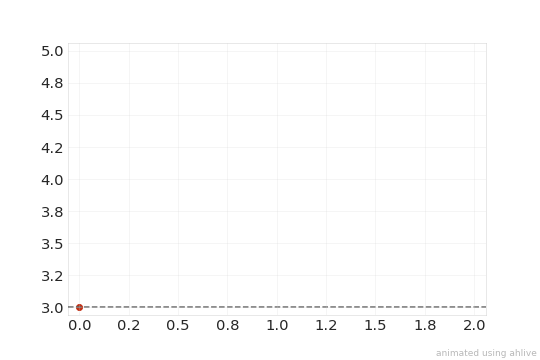## vertical span#

The reference chart changes depending on whether x0s, x1s, y0s, y1s is set.

If x0s and x1s is set, a vertical span is generated.

:

import ahlive as ah
arr = ah.Array([0, 1, 2], [3, 4, 5])
arr = arr.reference(x0s=[0, 1, 2], x1s=[0, 2, 4])
arr.render()

gifsicle: warning: trivial adaptive palette (only 220 colors in source)

: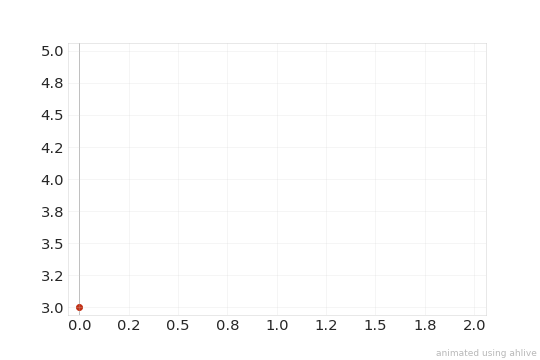## horizontal span#

Similarly if y0s and y1s is set, a horizontal span is generated.

:

import ahlive as ah
arr = ah.Array([0, 1, 2], [3, 4, 5])
arr = arr.reference(y0s=[3.25, 4, 4.5], y1s=[3, 4.5, 5])
arr.render()

gifsicle: warning: trivial adaptive palette (only 223 colors in source)

: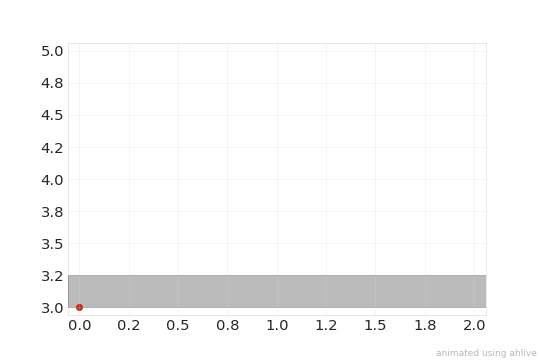## rectangular bound#

If all x0s, x1s, y0s, and y1s is set, a rectangle is generated.

:

import ahlive as ah
arr = ah.Array([0, 1, 2], [3, 4, 5])
arr = arr.reference(x0s=[0, 1, 2], x1s=[0, 2, 4], y0s=[3.25, 4, 4.5], y1s=[3, 4.5, 5])
arr.render()

gifsicle: warning: trivial adaptive palette (only 208 colors in source)

: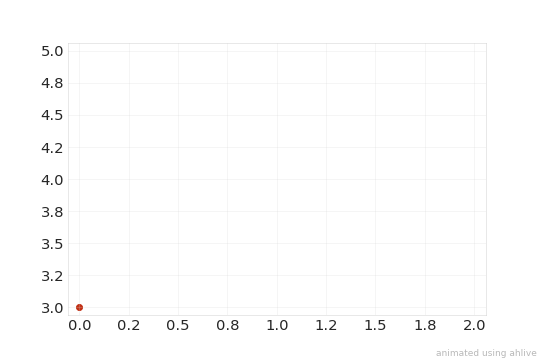## scatter point#

If only x0s and y0s is set, a point is generated.

:

import ahlive as ah
arr = ah.Array([0, 1, 2], [3, 4, 5])
arr = arr.reference(x0s=[0, 1, 2], y0s=[3.25, 4, 4.5])
arr.render()

gifsicle: warning: trivial adaptive palette (only 229 colors in source)

: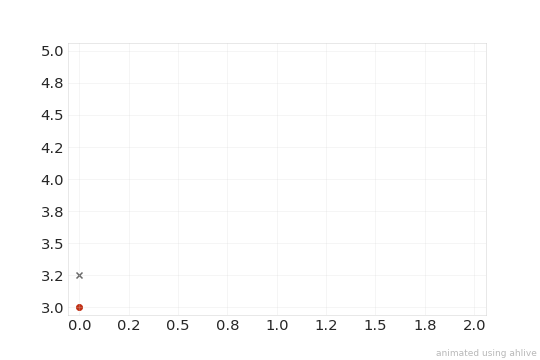## inline labels#

References also support inline_labels, but requires an inline_locs if the opposite axis is not set, i.e. y0s is not set when only x0s is set.

:

import ahlive as ah
arr = ah.Array([0, 1, 2], [3, 4, 5])
arr = arr.reference(
x0s=[0.25, 0.75, 1.75],
inline_locs=3.5,
inline_labels=['first', 'second', 'third']
)
arr.render()

gifsicle: warning: trivial adaptive palette (only 214 colors in source)

: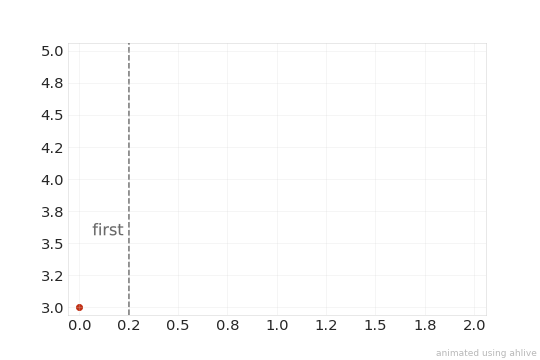## state labels#

Furthermore, references support state_labels.

:

import ahlive as ah
arr = ah.Array([0, 1, 2], [3, 4, 5])
arr = arr.reference(
x0s=[0.25, 0.75, 1.75],
state_labels=['a', 'bb', 'ccc']
)
arr.render()

gifsicle: warning: trivial adaptive palette (only 210 colors in source)

: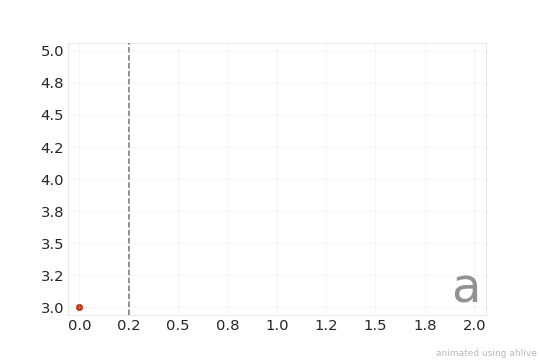## standalone reference#

If desired, references can be standalone by instantiating the class object, ah.Reference. The class works the same way; the only difference is that passing a label to substitute in the values is not possible, unless it’s overlaid with another object.

:

import ahlive as ah
ref = ah.Reference(
x0s=[0, 1],
inline_locs=0,
inline_labels=['A', 'B'],
state_labels=['d', 'e']
)
ref.render()

gifsicle: warning: trivial adaptive palette (only 218 colors in source)

: